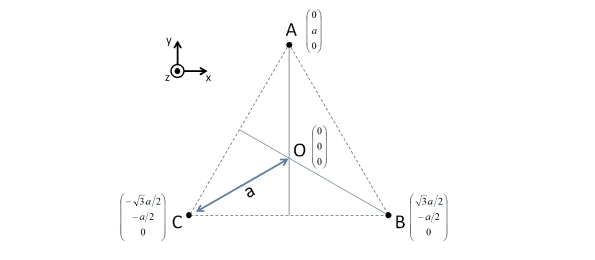# Electric field triangle

• various45
In summary, the conversation discusses solving an exercise involving three positive charges placed at the corners of an equilateral triangle and computing the electric field on the z-axis. The conversation also touches on the symmetry planes passing through each point on the axis and the value and direction of the electric field at the center of the triangle. The final question asks about the maximum electric field on the z-axis and its unique height above the triangle's center.

#### various45

Hi,

I'm trying to solve this exercize :

I/3 charges Q, positives, are at the corner of an equilateral triangle of centre O, in (x,O,y) . We want to compute the electric field created by the 3 charges on the axis z, passing by O and perpendicular at (x,O,y), thus computing the electric field E(M) in each M point of (Oz), located at an height z above O. We have OA=OB=OC.II/ 1) Describe the symmetry plane passing by each point M, located on the axis z. Tell the sense of direction of E(M) in each point of z.

As the point M is on axis z above A, I would say (Oz) is the only symmetry plane and the electric field is from A to the low triangle.

2) By a similar way, deduce the value of the electric field at the centre O

As the three charges are positives the vectorial sum of the 3 electric fields at point O would give an electric field whose value is O, but how could we deduce it by symmetry ?

3) Why would we have the electric field norm on the axis z created by the three charges reached a maximum at a unique height above O ?

I do not see how to answer this one.

Thank you very much

Hello Various (!?) , welcome to PF!

As the point M is on axis z above A, I would say (Oz) is the only symmetry plane and the electric field is from A to the low triangle.

Oz is a line, not a plane !

By a similar way, deduce the value of the electric field at the centre O
If you have brought 1) to a good conclusion, this one will be easier. There is one more symmetry plane for O

I do not see how to answer this one (3)
Again, this will be easier once we have 1) and 2).

indeed the only symmetry plane would be (ux,uz) but how could we deduve the sense according to this plane ?

Well, if, with (ux,uz) you mean the x-z plane, I don't agree !
Applying this 'symmetry' would flip the picture upside down and then it isn't the same as the original !

I mean the plane which splits in two the triangle from bottom to top vertically but I do not know how to write it, until now I was doing electric field in 3D with cylindric or spheric so it was also the same planes, here we have a triangle so I would say if we take cylindric coordinate (r; theta, z) the plane (r,z) splits vertically the triangle and it is the same

various45 said:
Hi,

I'm trying to solve this exercize :

I/3 charges Q, positives, are at the corner of an equilateral triangle of centre O, in (x,O,y) . We want to compute the electric field created by the 3 charges on the axis z, passing by O and perpendicular at (x,O,y), thus computing the electric field E(M) in each M point of (Oz), located at an height z above O. We have OA=OB=OC.II/ 1) Describe the symmetry plane passing by each point M, located on the axis z. Tell the sense of direction of E(M) in each point of z.

As the point M is on axis z above A, I would say (Oz) is the only symmetry plane and the electric field is from A to the low triangle.

2) By a similar way, deduce the value of the electric field at the centre O

As the three charges are positives the vectorial sum of the 3 electric fields at point O would give an electric field whose value is O, but how could we deduce it by symmetry ?

3) Why would we have the electric field norm on the axis z created by the three charges reached a maximum at a unique height above O ?

I do not see how to answer this one.

Thank you very much
Are you certain that you have a equilateral triangle?

Maybe I'm having a brain cramp, but it doesn't seem to have three sides of equal length.